# Force Force Any push or pull exerted on

• Slides: 91ForceForce • Any push or pull exerted on an objectSystem • The object with the force appliedEnvironment • The world surrounding the objectContact Force • A force that acts on an object by touching itContact Force • A baseball bat striking a ballLong-range Force • A force that acts on an object w/o touching it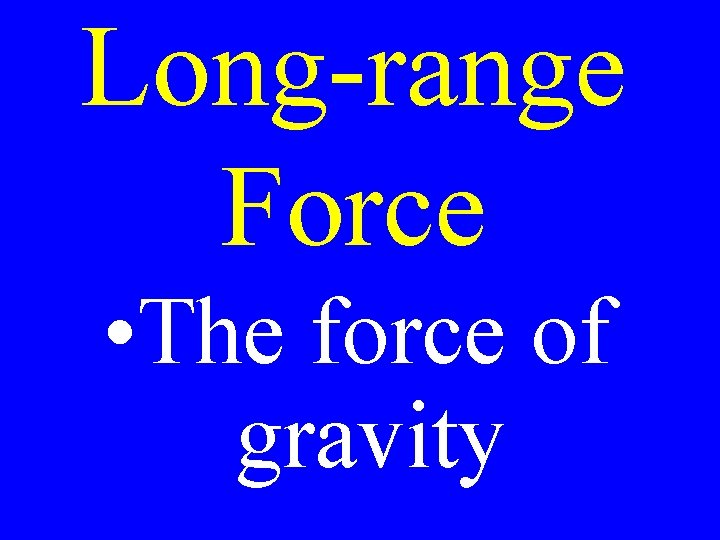Long-range Force • The force of gravityAgent • Whatever is causing the forceInertia • The resistance to change (in motion)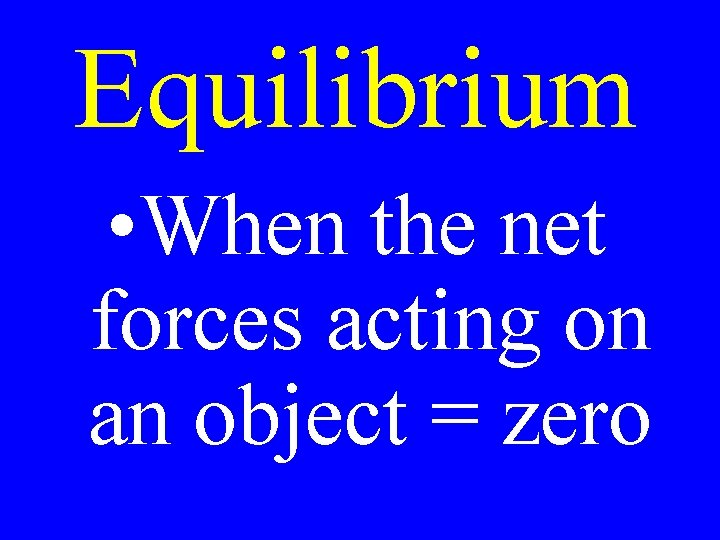Equilibrium • When the net forces acting on an object = zeroForce Vector Diagram • A Diagram showing the vectors of all forces acting on an object.Force Vector Diagram Force of table on the ball Weight on ballDraw Force Vector Diagrams of: 1)A book on a desk 2)A book being pushed across the desk 3)A book fallingNewton’s Laws of Motion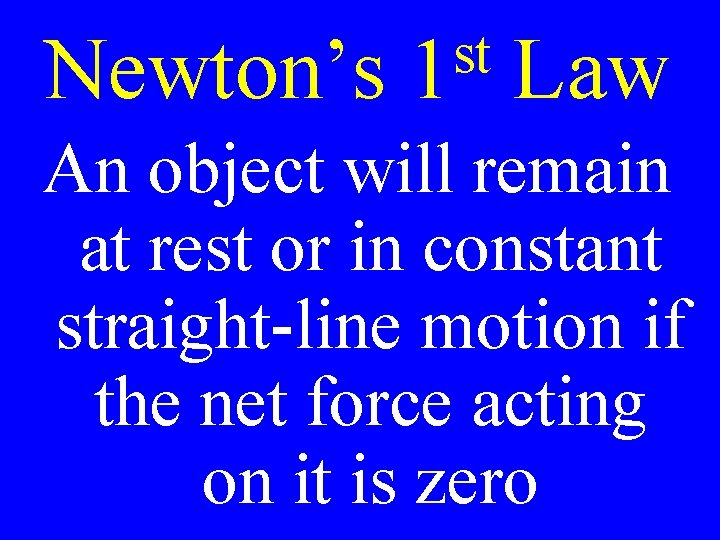Newton’s st 1 Law An object will remain at rest or in constant straight-line motion if the net force acting on it is zero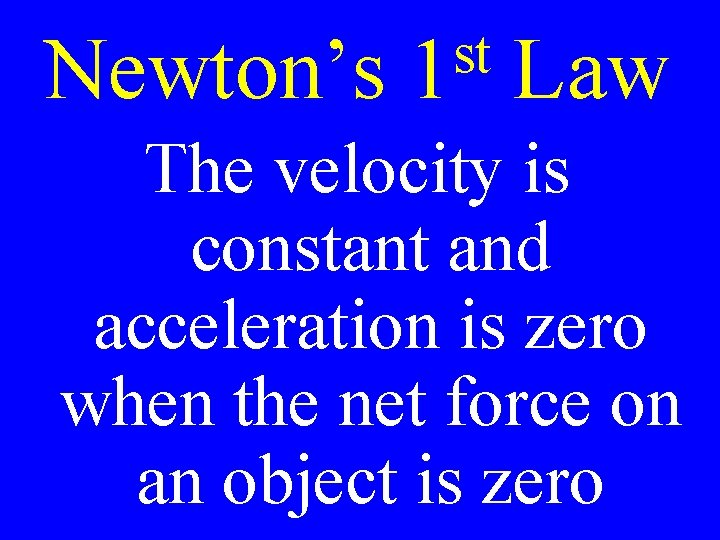Newton’s st 1 Law The velocity is constant and acceleration is zero when the net force on an object is zero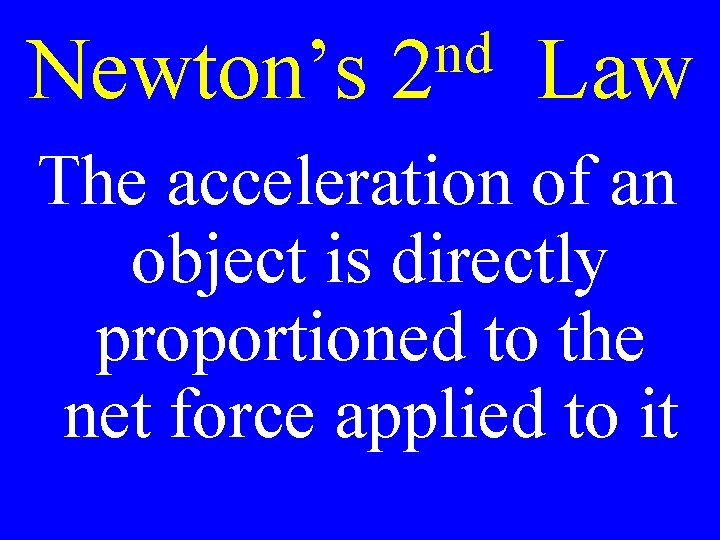Newton’s nd 2 Law The acceleration of an object is directly proportioned to the net force applied to itNewton’s a= nd 2 Fnet m LawNewton’s nd 2 Law Fnet = ma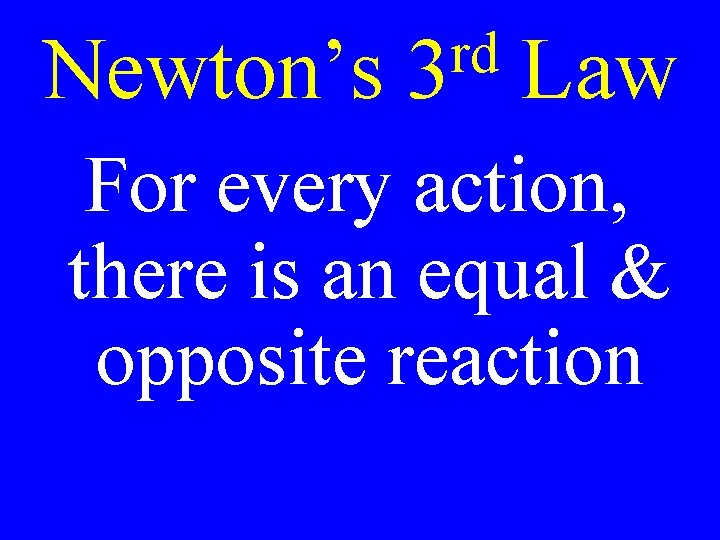Newton’s rd 3 Law For every action, there is an equal & opposite reaction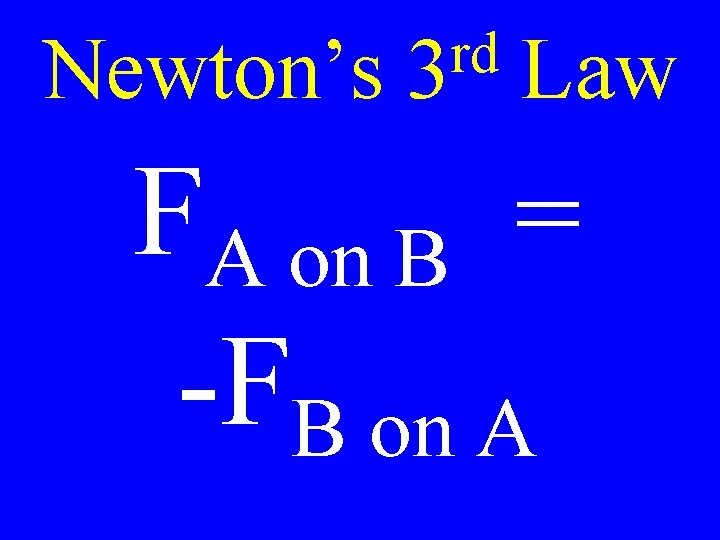Newton’s rd 3 Law FA on B = -FB on A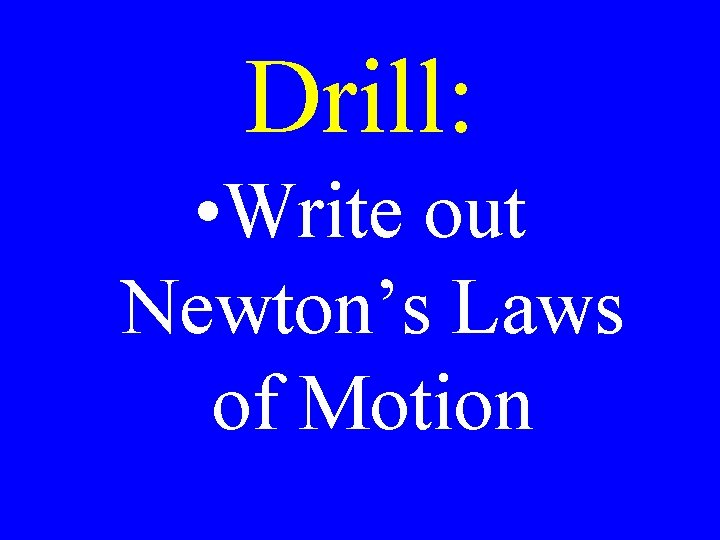Drill: • Write out Newton’s Laws of Motion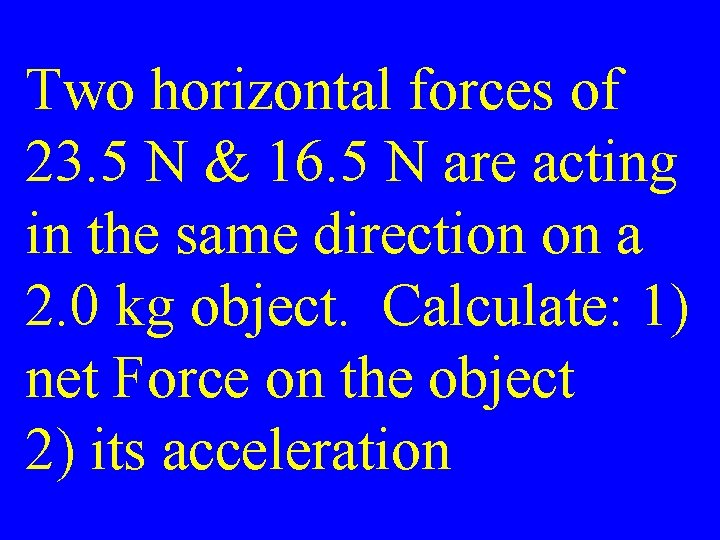Two horizontal forces of 23. 5 N & 16. 5 N are acting in the same direction on a 2. 0 kg object. Calculate: 1) net Force on the object 2) its acceleration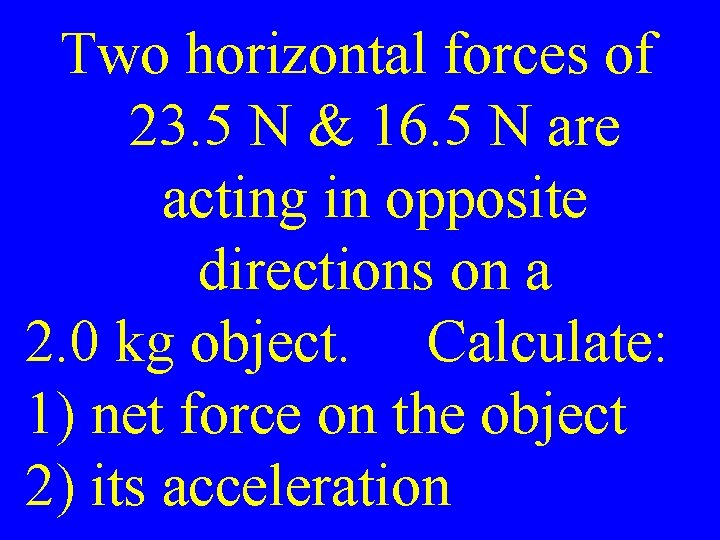Two horizontal forces of 23. 5 N & 16. 5 N are acting in opposite directions on a 2. 0 kg object. Calculate: 1) net force on the object 2) its acceleration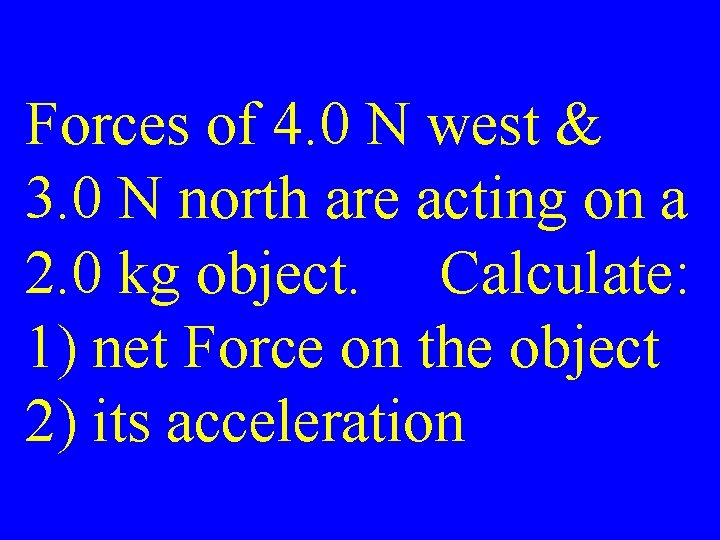Forces of 4. 0 N west & 3. 0 N north are acting on a 2. 0 kg object. Calculate: 1) net Force on the object 2) its acceleration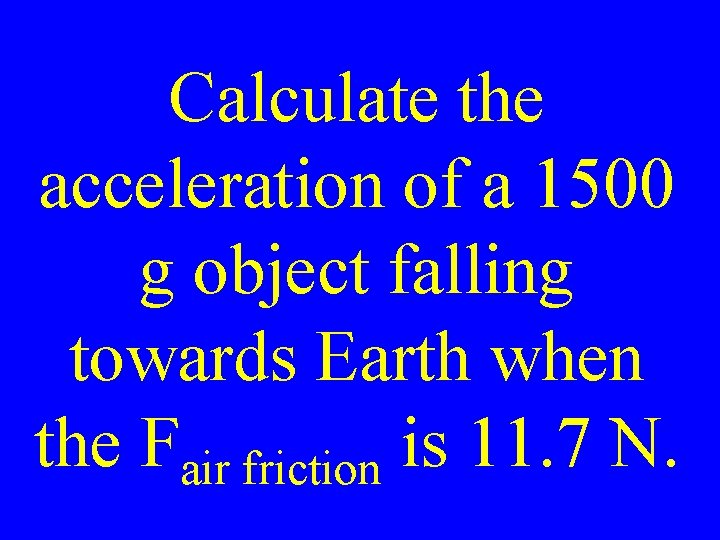Calculate the acceleration of a 1500 g object falling towards Earth when the Fair friction is 11. 7 N.List Newton’s Laws of MotionTypes of Forces Friction Normal Spring Tension Thrust Weight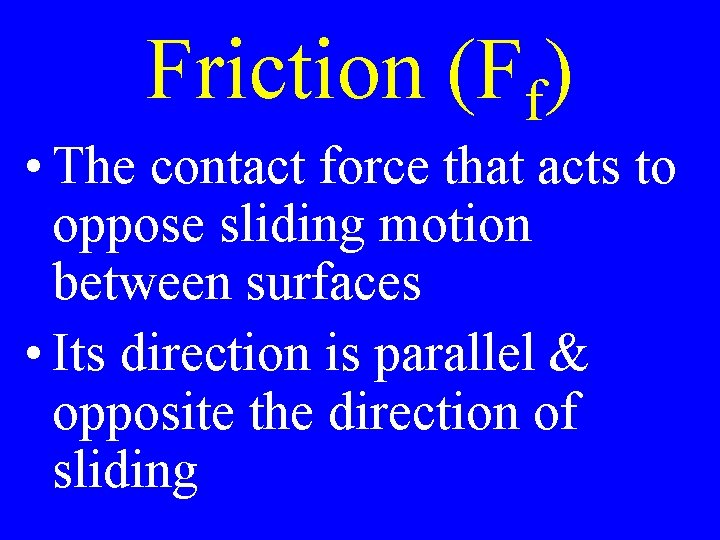Friction (Ff) • The contact force that acts to oppose sliding motion between surfaces • Its direction is parallel & opposite the direction of slidingNormal (FN) • The contact force exerted by a surface on an object • Its direction is perpendicular & away from the surface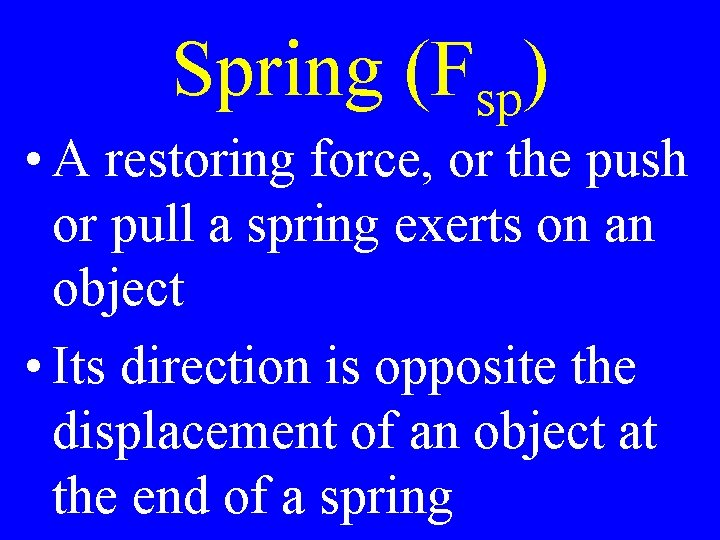Spring (Fsp) • A restoring force, or the push or pull a spring exerts on an object • Its direction is opposite the displacement of an object at the end of a springTension (FT) • The pull exerted by a string, rope, or cable when attached to a body & pulled taut • Its direction away from the object & parallel to the string at the point of attachment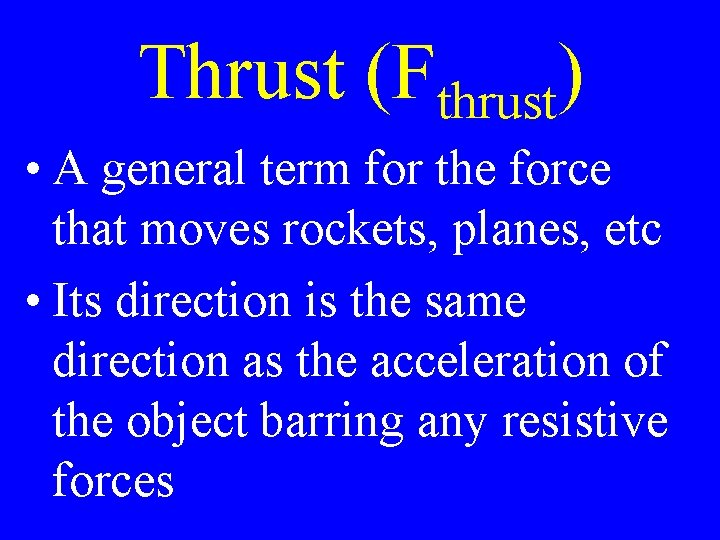Thrust (Fthrust) • A general term for the force that moves rockets, planes, etc • Its direction is the same direction as the acceleration of the object barring any resistive forcesWeight (Fg) • Force due the gravitational attraction between two objects like an object & the Earth • Its direction is straight down towards the center of the Earth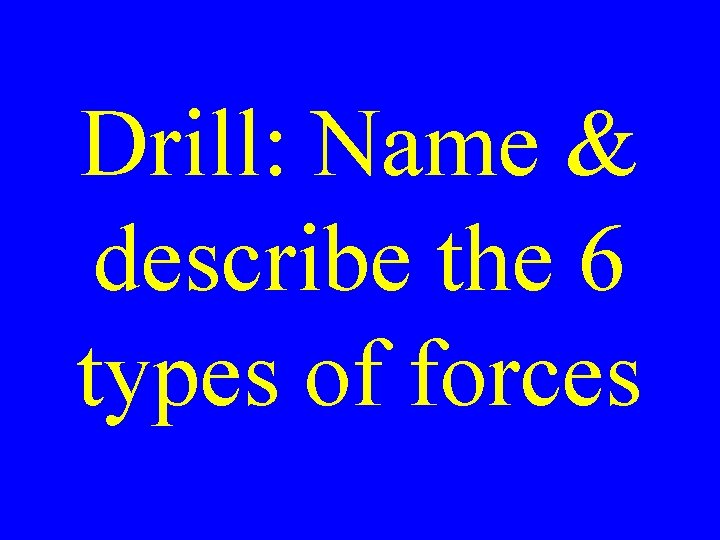Drill: Name & describe the 6 types of forcesWeight (Fg) Weight = Fg = mag = mg Fg = W = mgWhen an object is launched, the only forces acting upon it are the forces gravity & air friction.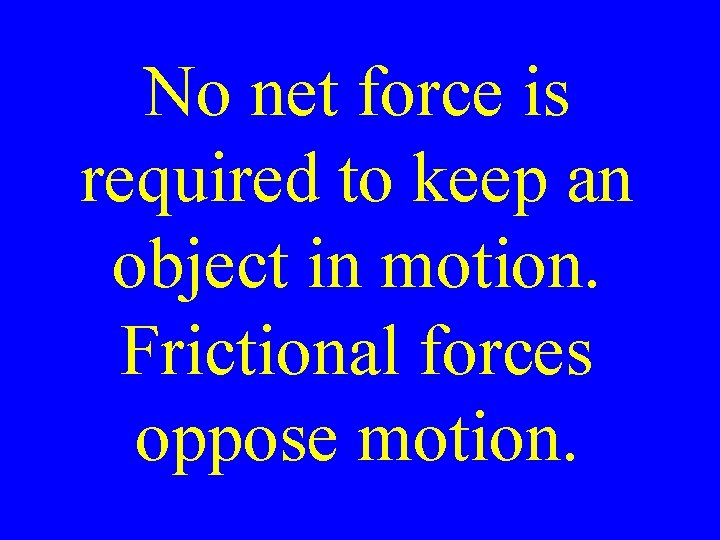No net force is required to keep an object in motion. Frictional forces oppose motion.Inertia is not a force, but the resistance to the change in motion or momentum.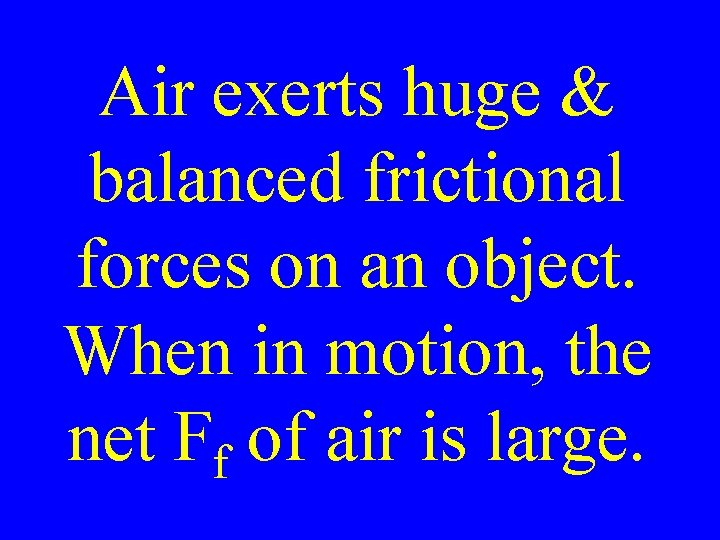Air exerts huge & balanced frictional forces on an object. When in motion, the net Ff of air is large.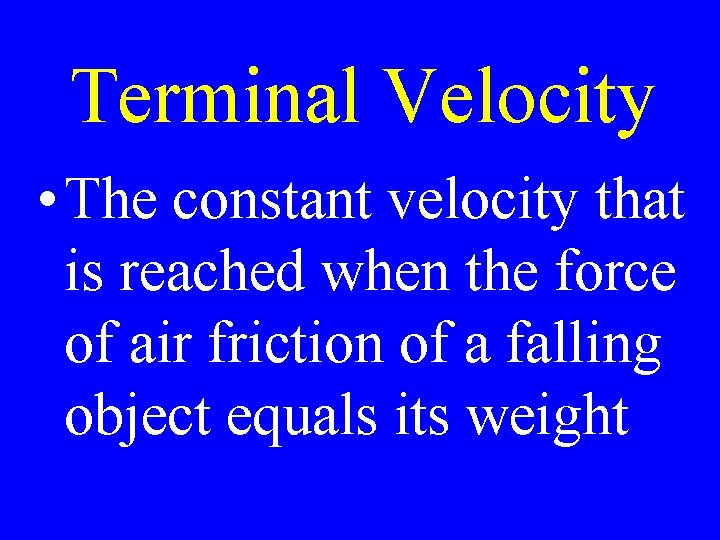Terminal Velocity • The constant velocity that is reached when the force of air friction of a falling object equals its weight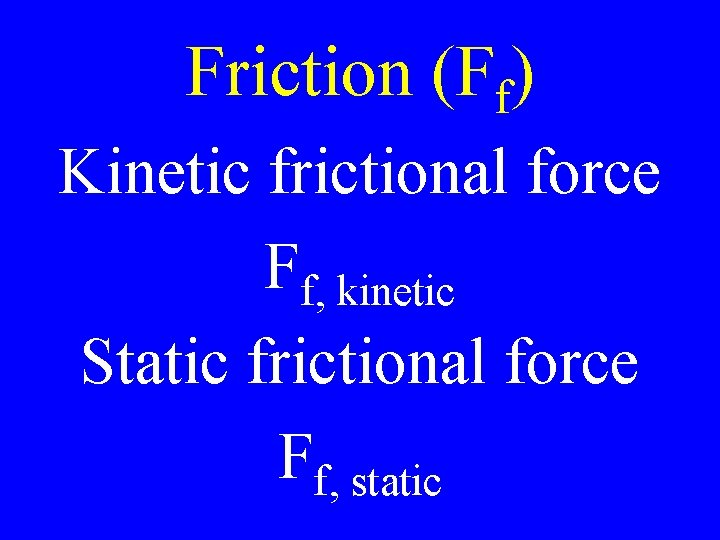Friction (Ff) Kinetic frictional force Ff, kinetic Static frictional force Ff, static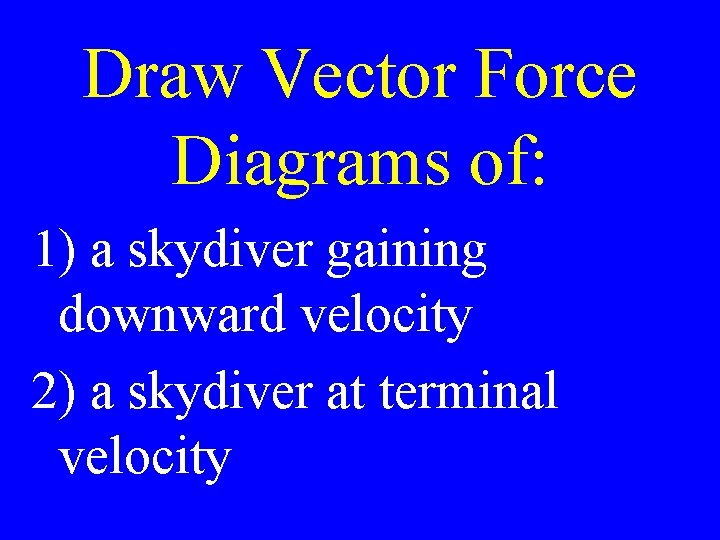Draw Vector Force Diagrams of: 1) a skydiver gaining downward velocity 2) a skydiver at terminal velocity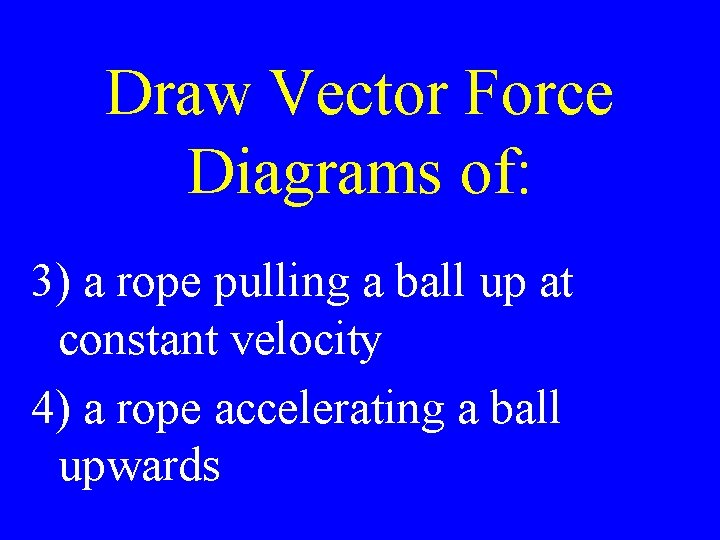Draw Vector Force Diagrams of: 3) a rope pulling a ball up at constant velocity 4) a rope accelerating a ball upwards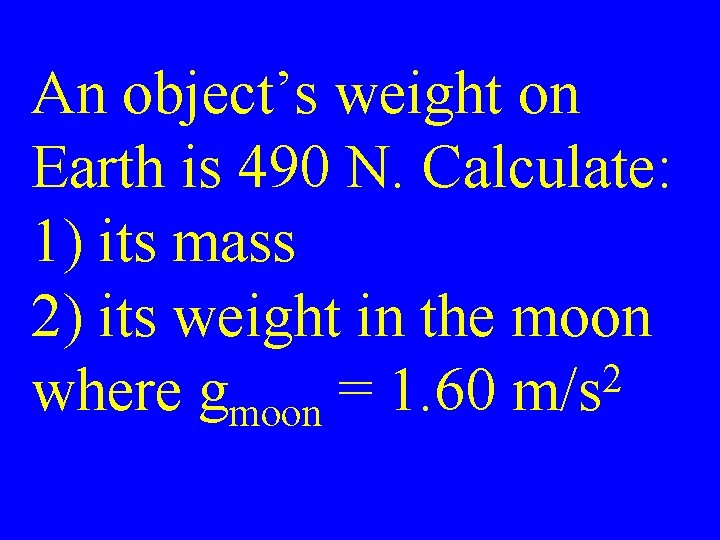An object’s weight on Earth is 490 N. Calculate: 1) its mass 2) its weight in the moon 2 where gmoon = 1. 60 m/s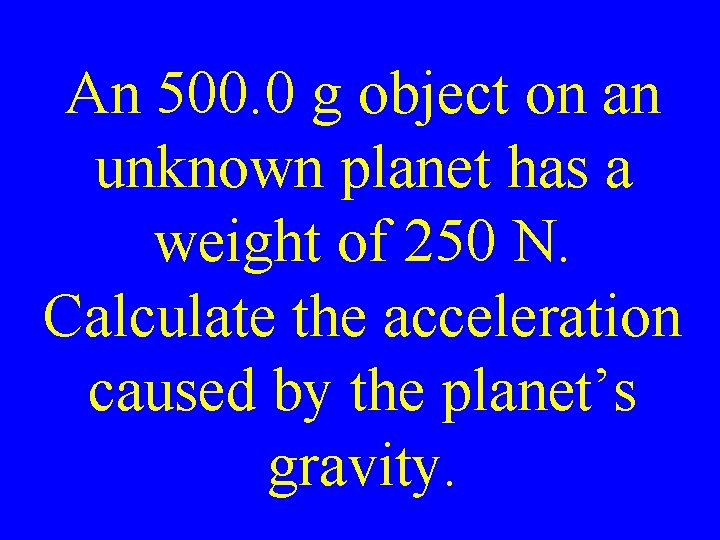An 500. 0 g object on an unknown planet has a weight of 250 N. Calculate the acceleration caused by the planet’s gravity.Static Ff • The force exerted on one surface by another when there is no relative motionKinetic Ff • The force exerted on one surface by another when in relative motion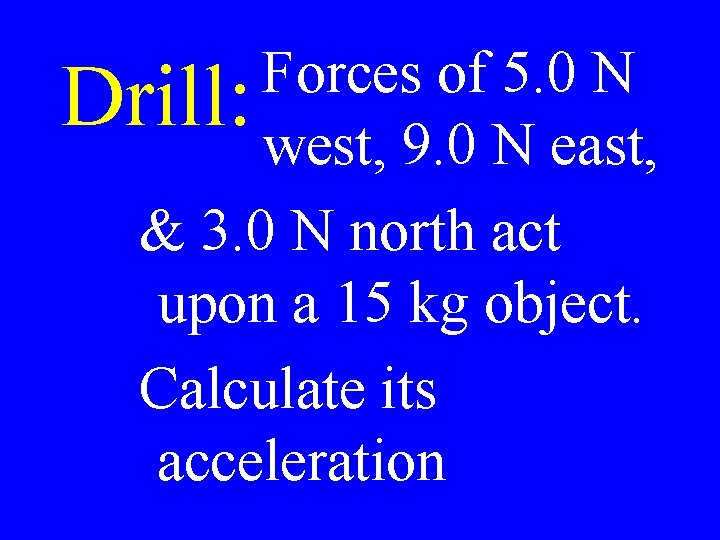Forces of 5. 0 N Drill: west, 9. 0 N east, & 3. 0 N north act upon a 15 kg object. Calculate its accelerationForces acting on an object: FN = -W FA > F f FN Ff Fg or Weight Fapplied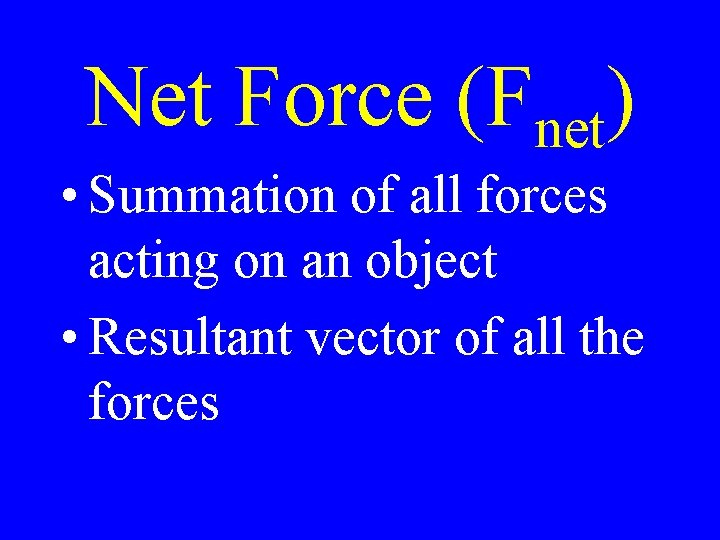Net Force (Fnet) • Summation of all forces acting on an object • Resultant vector of all the forces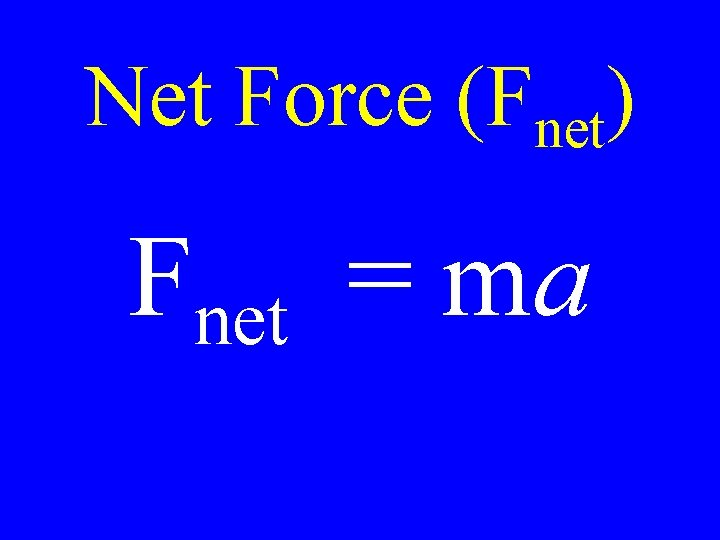Net Force (Fnet) Fnet = ma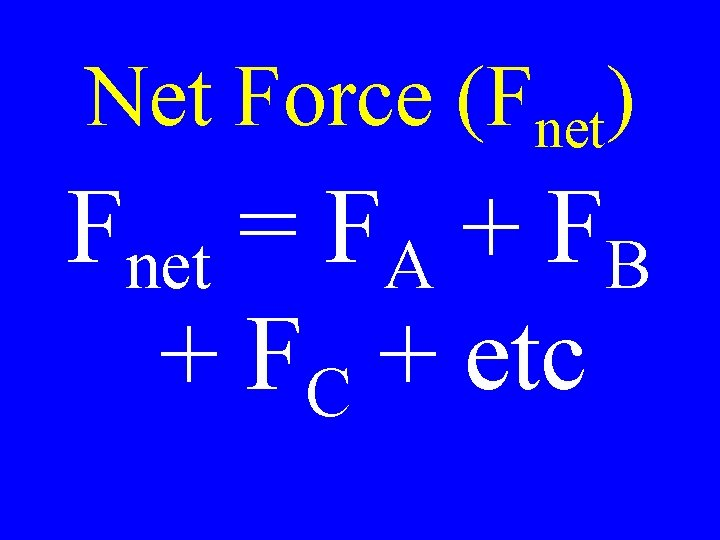Net Force (Fnet) Fnet = FA + FB + FC + etc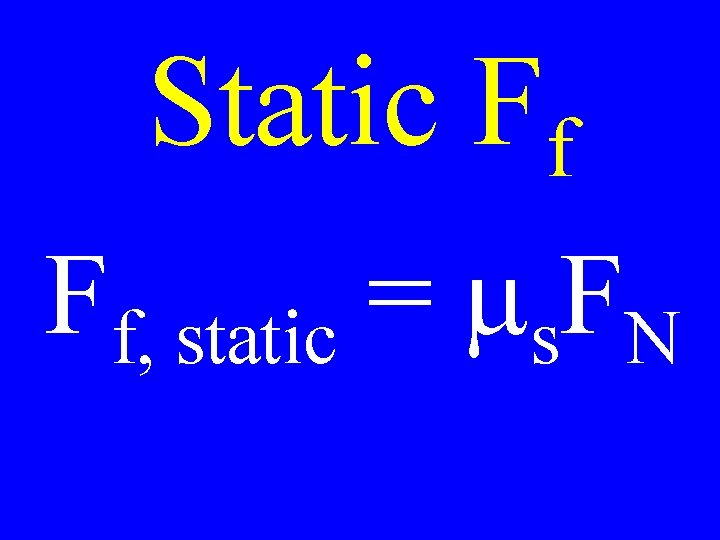Static Ff Ff, static = ms. FN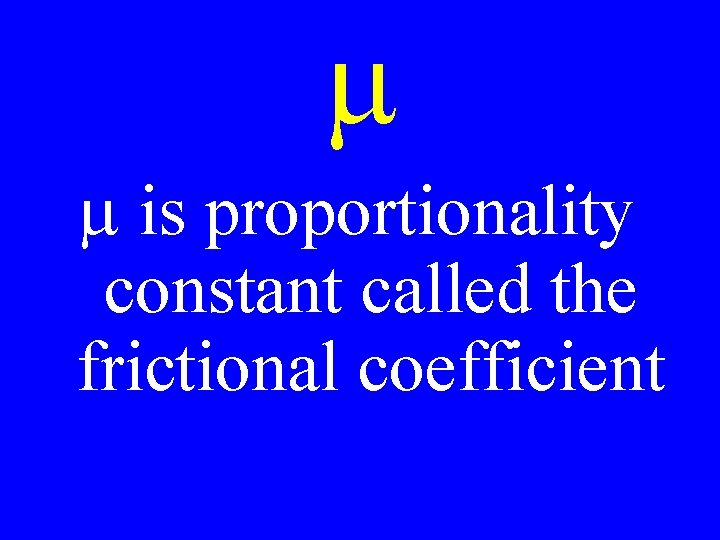m m is proportionality constant called the frictional coefficient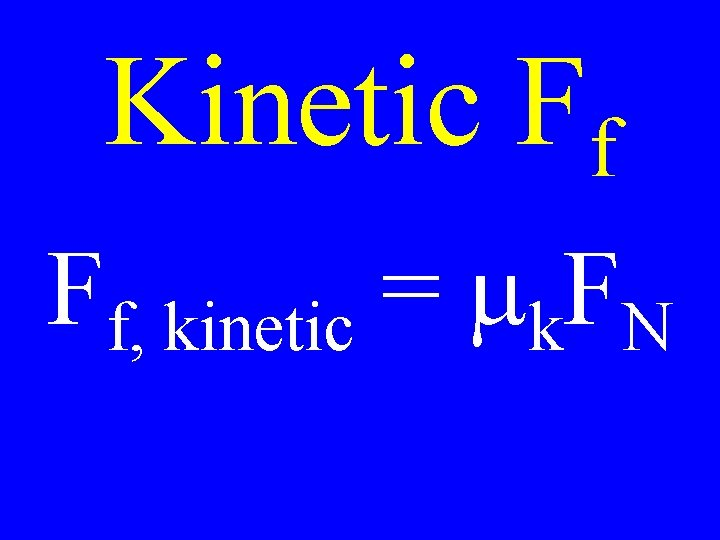Kinetic Ff Ff, kinetic = mk. FN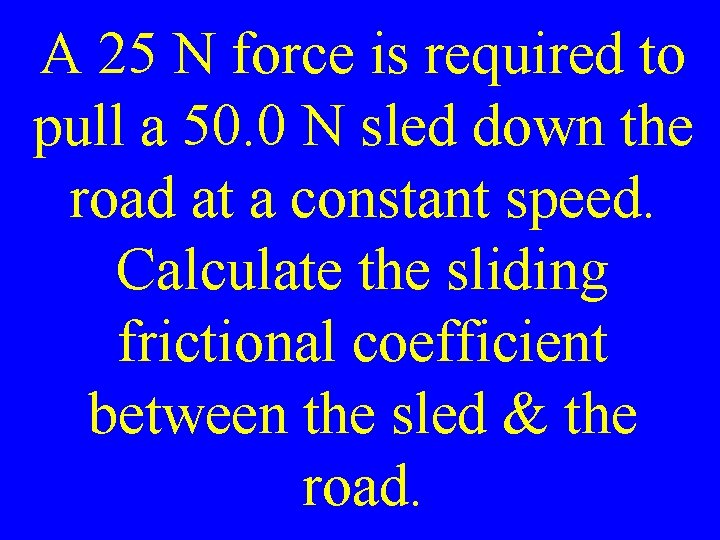A 25 N force is required to pull a 50. 0 N sled down the road at a constant speed. Calculate the sliding frictional coefficient between the sled & the road.A person & a sled have a total weight of 490 N. The sliding frictional coefficient between the sled & the snow is 0. 10. Calculate the force required to pull the sled at constant speed.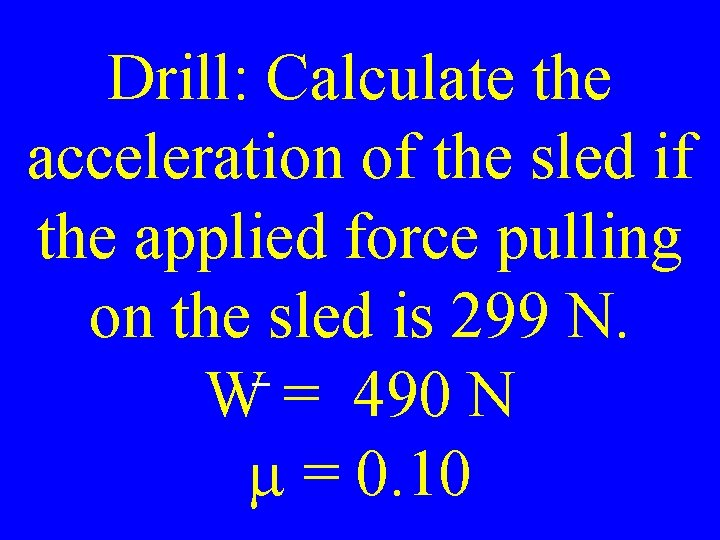Drill: Calculate the acceleration of the sled if the applied force pulling on the sled is 299 N. W = 490 N m = 0. 10Calculate the force required to pull a 500. 0 g block with an 2 acceleration of 3. 0 m/s. m = 0. 50Periodic Motion • Repetitive or vibrational motion like that of a spring, swing or pendulum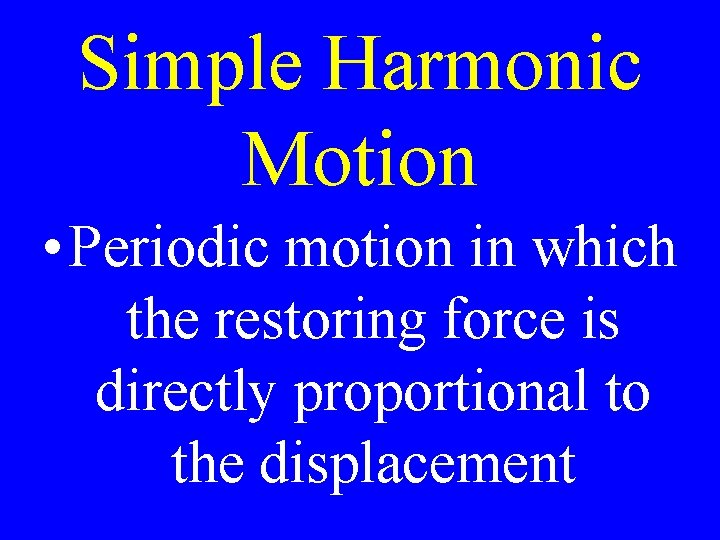Simple Harmonic Motion • Periodic motion in which the restoring force is directly proportional to the displacement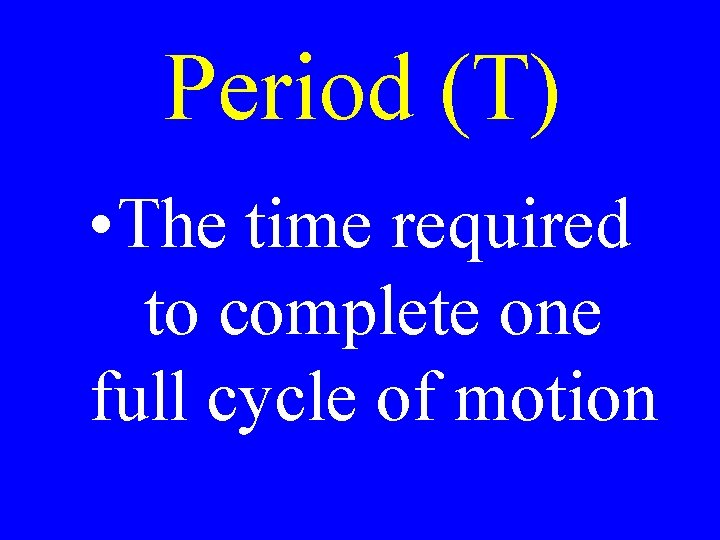Period (T) • The time required to complete one full cycle of motionAmplitude • Maximum displacement from the zero point or equilibriumPendulum Motion Formula l T = 2 p ---agCalculate the period of a pendulum with a length of 49 cm:Drill: Calculate the length of the pendulum of a grandfather clock whose period is equal 1. 0 second: C HW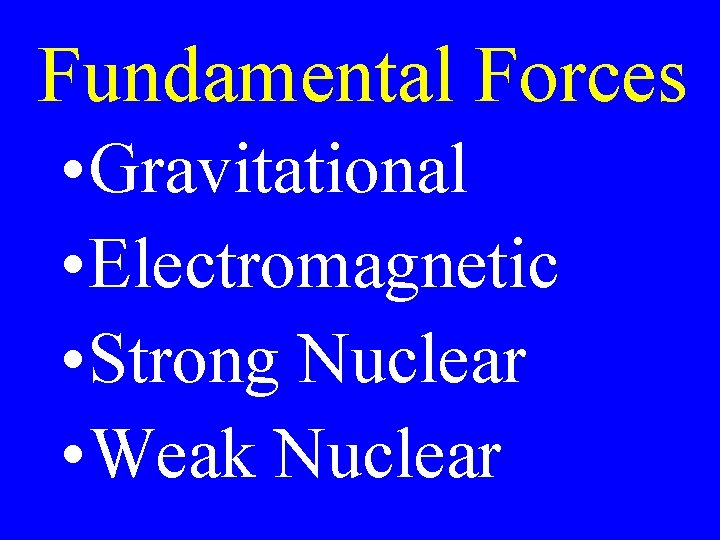Fundamental Forces • Gravitational • Electromagnetic • Strong Nuclear • Weak Nuclear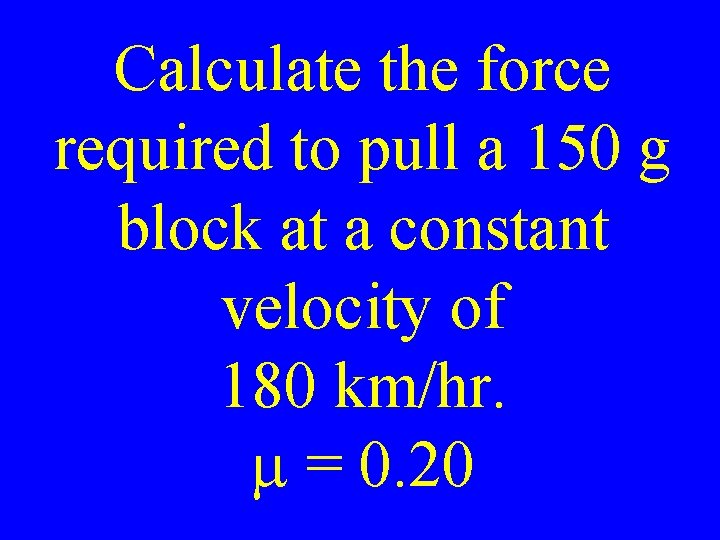Calculate the force required to pull a 150 g block at a constant velocity of 180 km/hr. m = 0. 20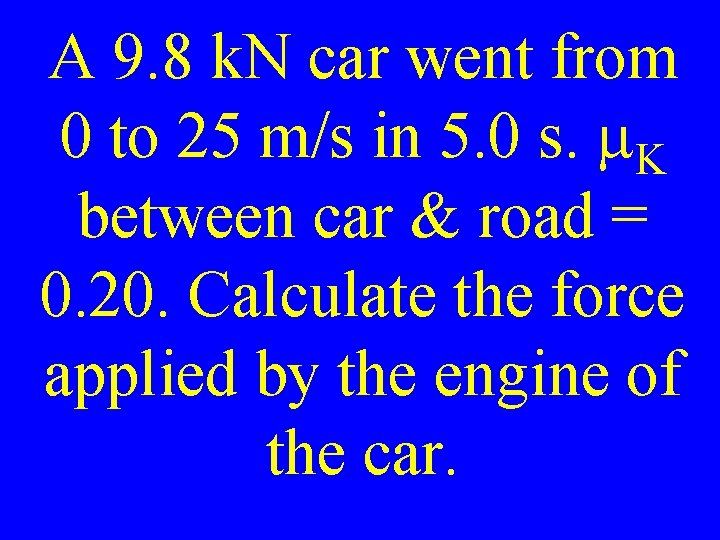A 9. 8 k. N car went from 0 to 25 m/s in 5. 0 s. m. K between car & road = 0. 20. Calculate the force applied by the engine of the car.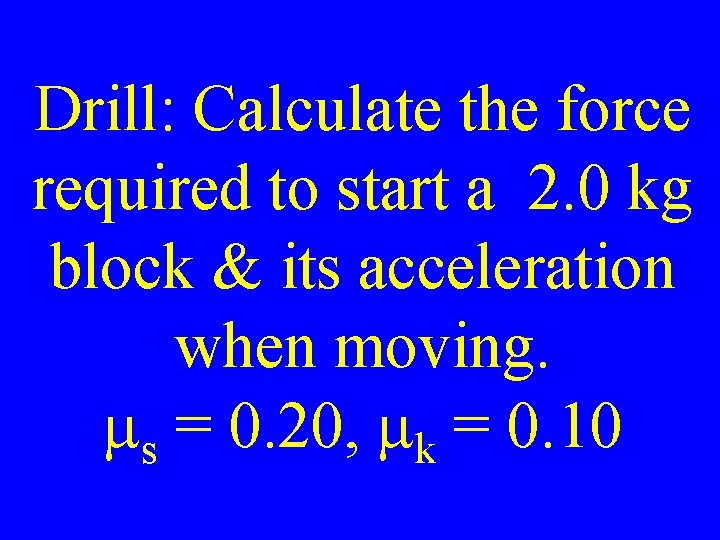Drill: Calculate the force required to start a 2. 0 kg block & its acceleration when moving. ms = 0. 20, mk = 0. 10Calculate the force required to start a 2. 0 kg block & calculate its acceleration when moving. ms = 0. 20, mk = 0. 10A 6. 0 kg ball is attached by a rope over a pulley to a 4. 0 kg ball. 1) Draw the problem. 2) Calculate each ball’s acceleration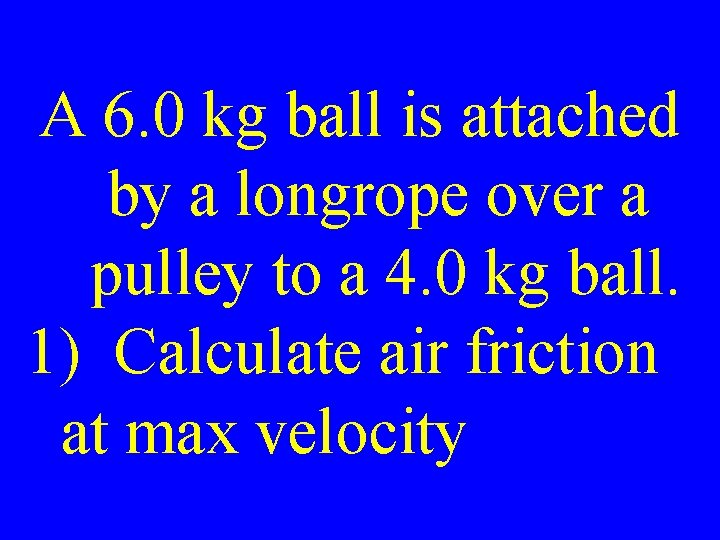A 6. 0 kg ball is attached by a longrope over a pulley to a 4. 0 kg ball. 1) Calculate air friction at max velocity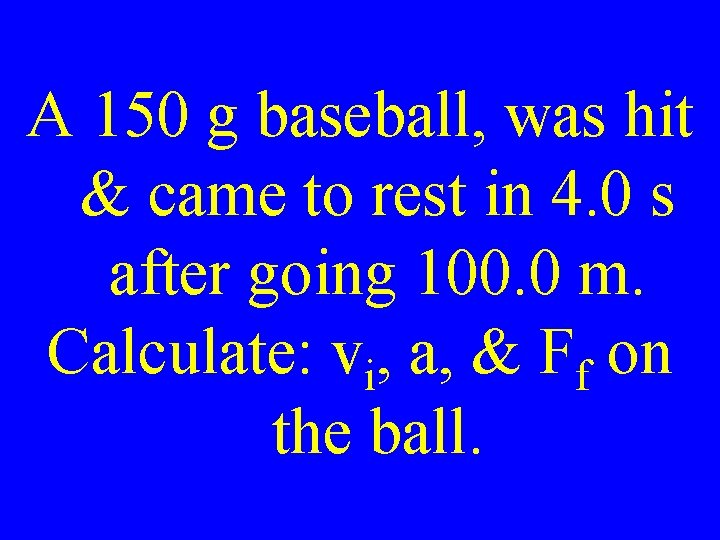A 150 g baseball, was hit & came to rest in 4. 0 s after going 100. 0 m. Calculate: vi, a, & Ff on the ball.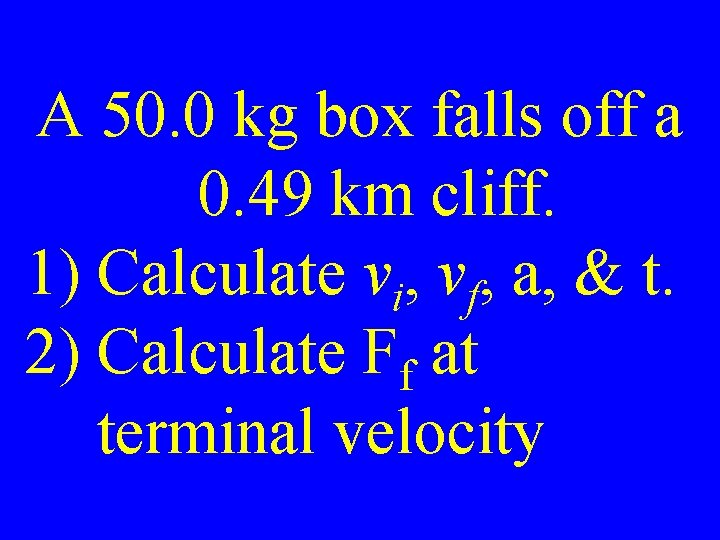A 50. 0 kg box falls off a 0. 49 km cliff. 1) Calculate vi, vf, a, & t. 2) Calculate Ff at terminal velocityA 10. 0 kg box falls off a 0. 49 km cliff & hits the ground in 20. 0 s. 1) Calculate vf & a. 2) Calculate Ff if air friction is included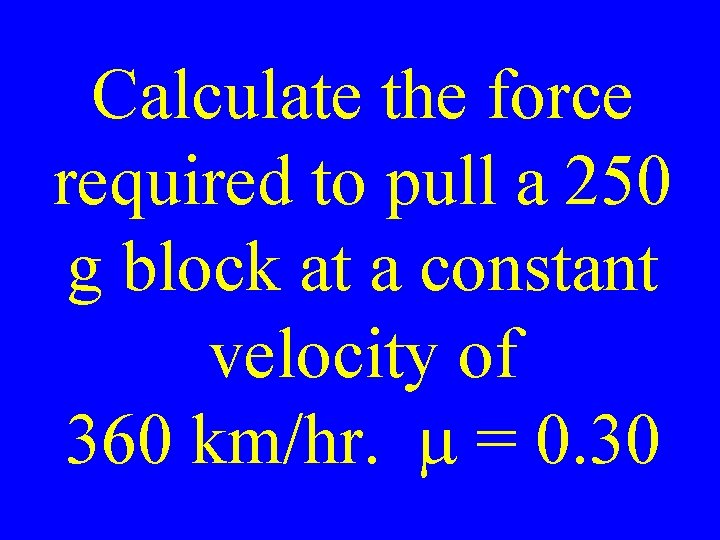Calculate the force required to pull a 250 g block at a constant velocity of 360 km/hr. m = 0. 30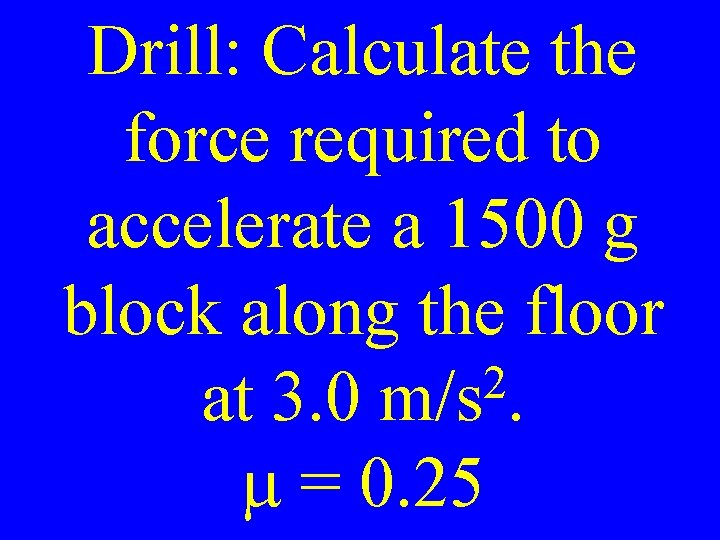Drill: Calculate the force required to accelerate a 1500 g block along the floor 2 at 3. 0 m/s. m = 0. 25A 65 kg boy & a 35 kg girl are in a tug-of-war on ice. The girl’s acceleration is 13 2 cm/s. Calculate the boy’s acceleration.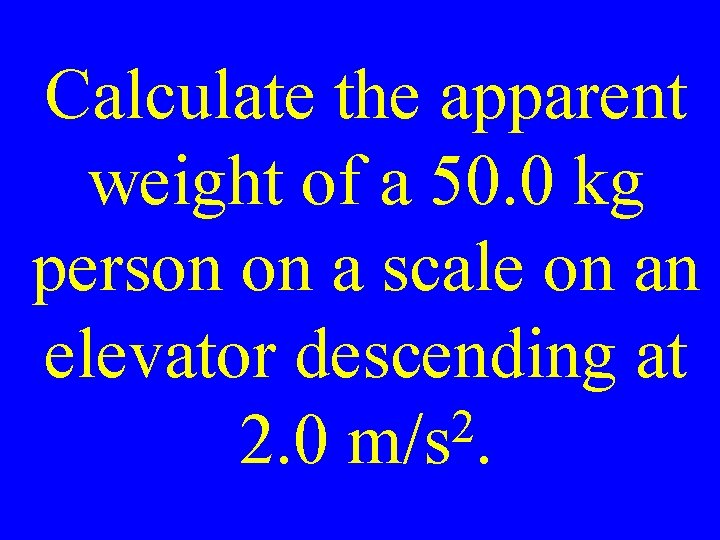Calculate the apparent weight of a 50. 0 kg person on a scale on an elevator descending at 2 2. 0 m/s.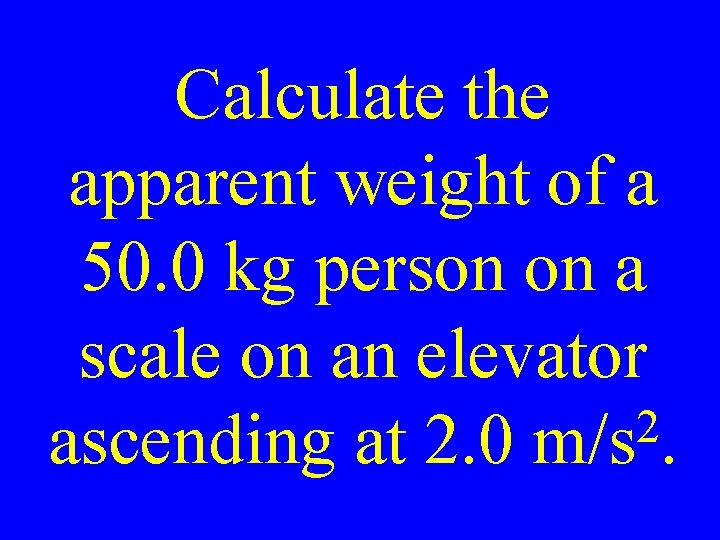Calculate the apparent weight of a 50. 0 kg person on a scale on an elevator 2 ascending at 2. 0 m/s.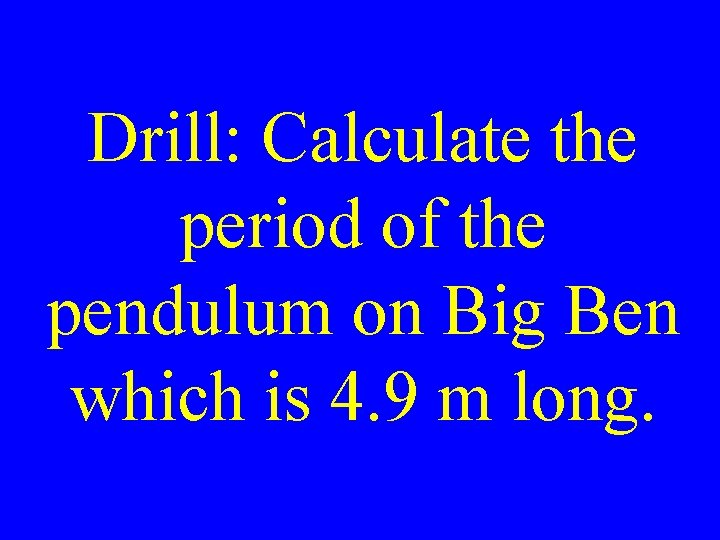Drill: Calculate the period of the pendulum on Big Ben which is 4. 9 m long.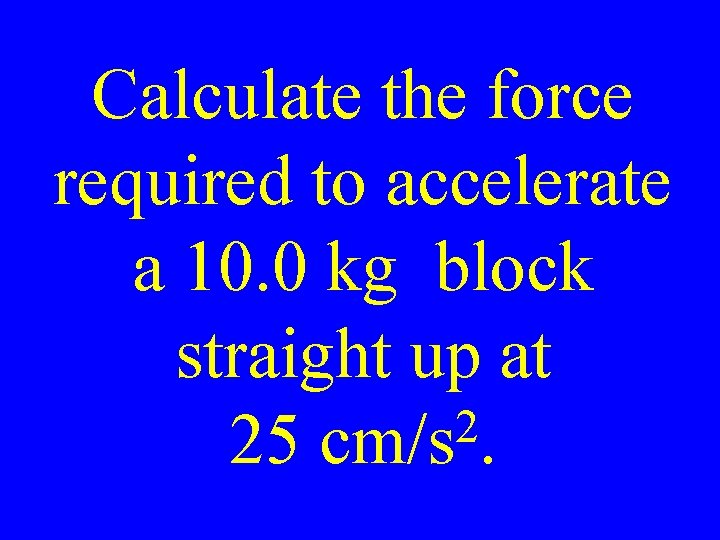Calculate the force required to accelerate a 10. 0 kg block straight up at 2 25 cm/s.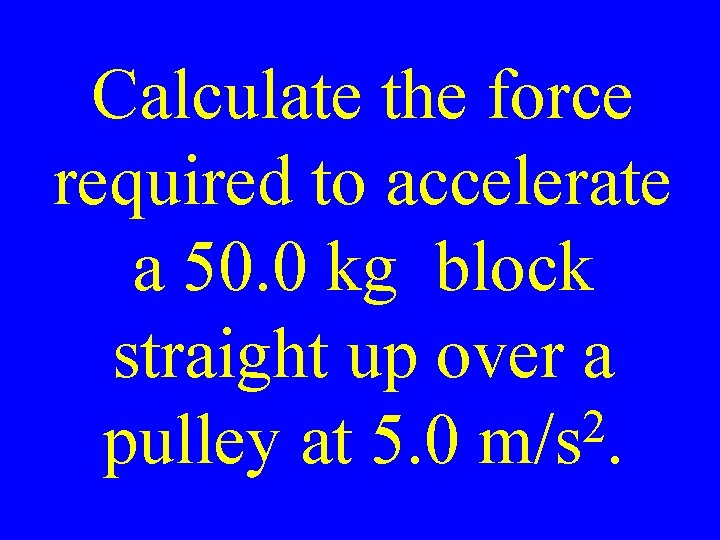Calculate the force required to accelerate a 50. 0 kg block straight up over a 2 pulley at 5. 0 m/s.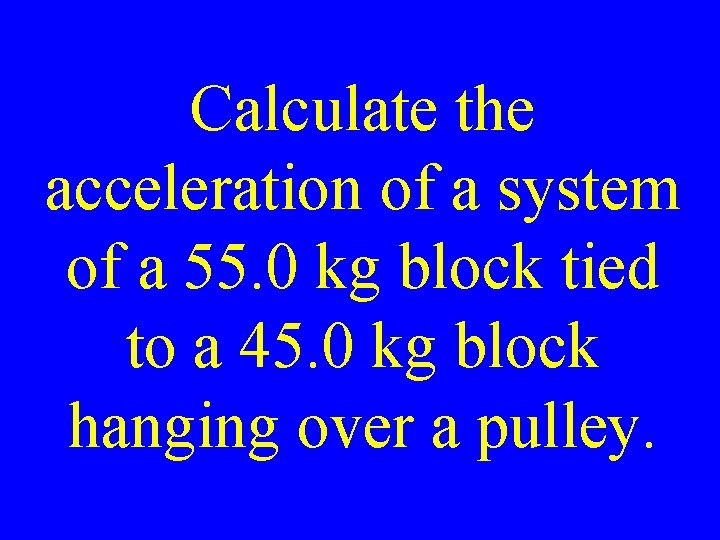Calculate the acceleration of a system of a 55. 0 kg block tied to a 45. 0 kg block hanging over a pulley.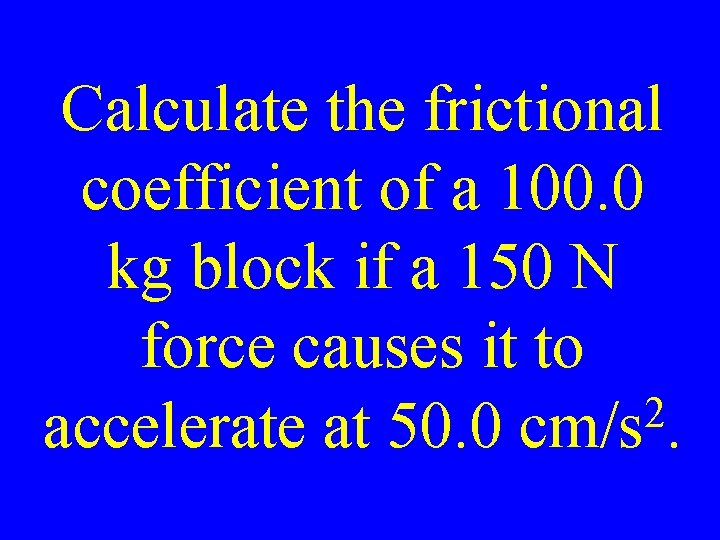Calculate the frictional coefficient of a 100. 0 kg block if a 150 N force causes it to 2 accelerate at 50. 0 cm/s.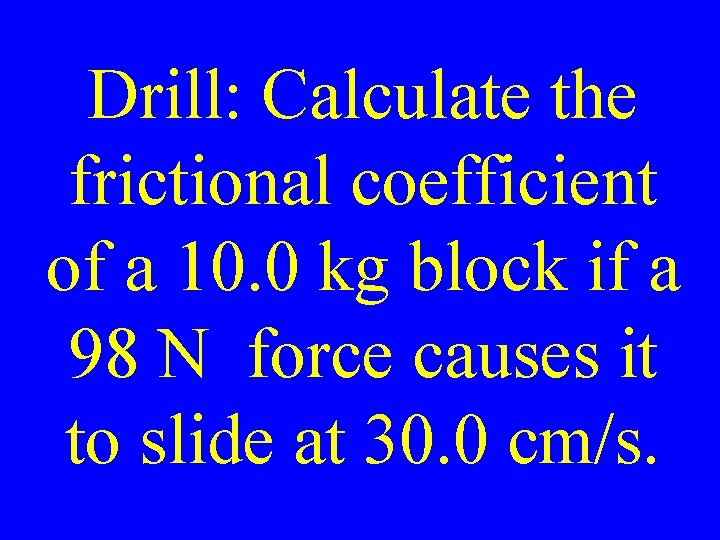Drill: Calculate the frictional coefficient of a 10. 0 kg block if a 98 N force causes it to slide at 30. 0 cm/s.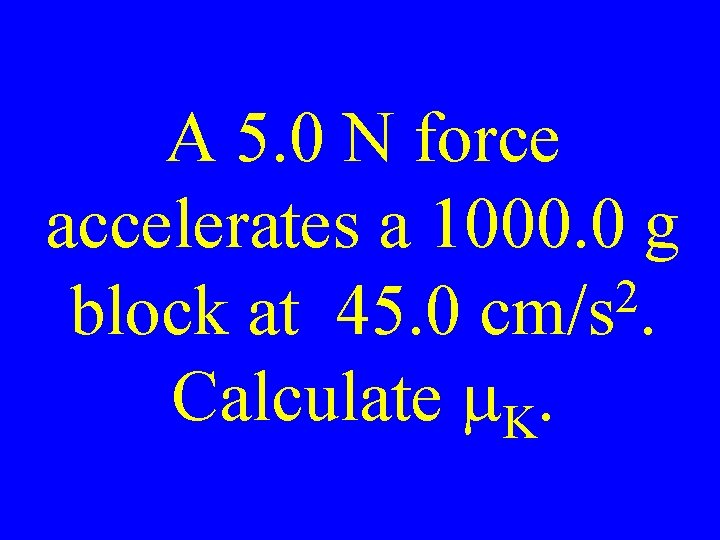A 5. 0 N force accelerates a 1000. 0 g 2 block at 45. 0 cm/s. Calculate m. K.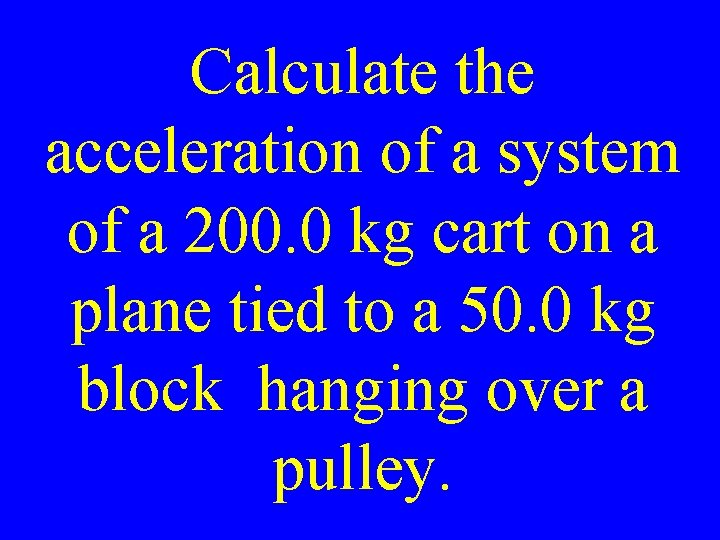Calculate the acceleration of a system of a 200. 0 kg cart on a plane tied to a 50. 0 kg block hanging over a pulley.